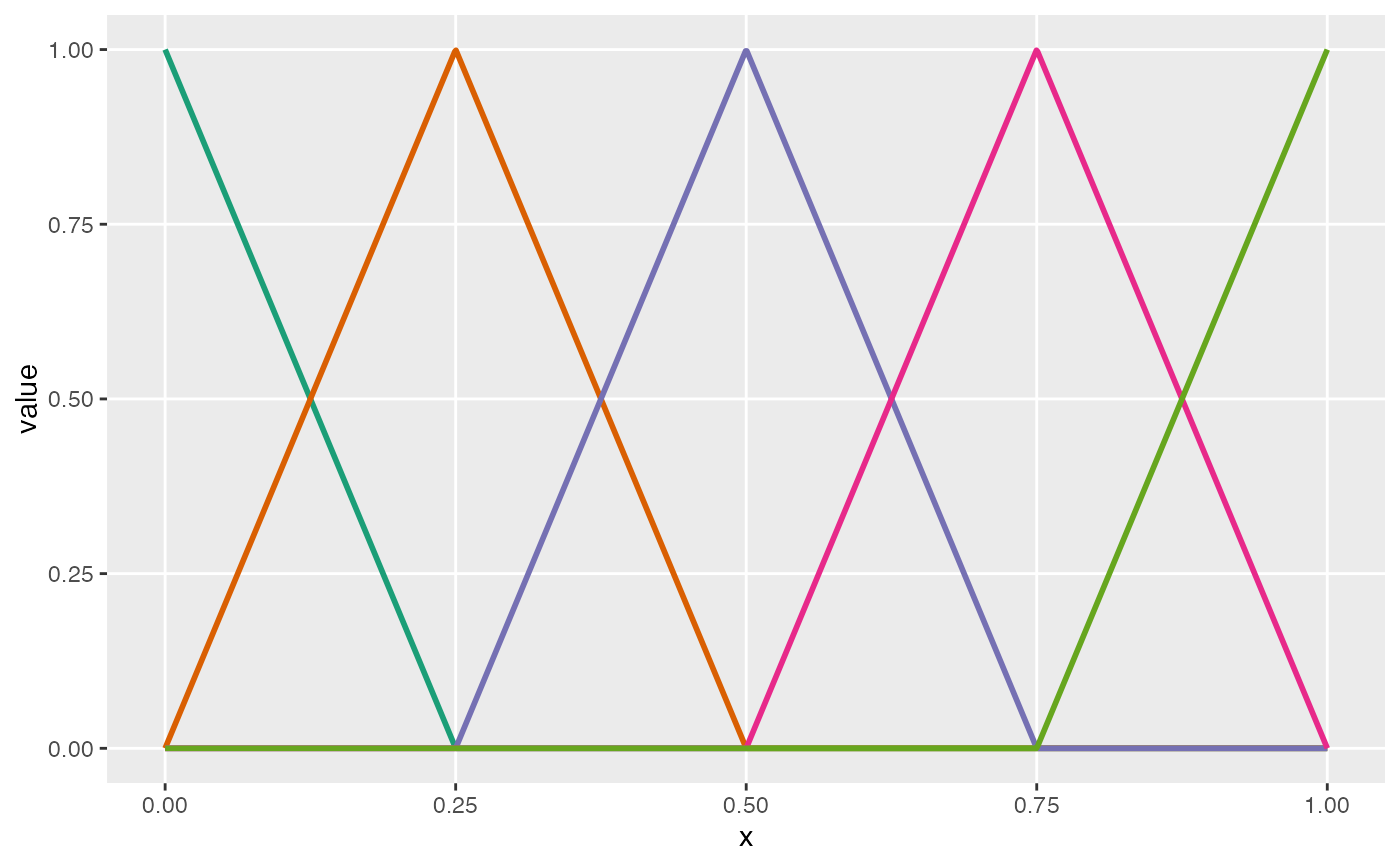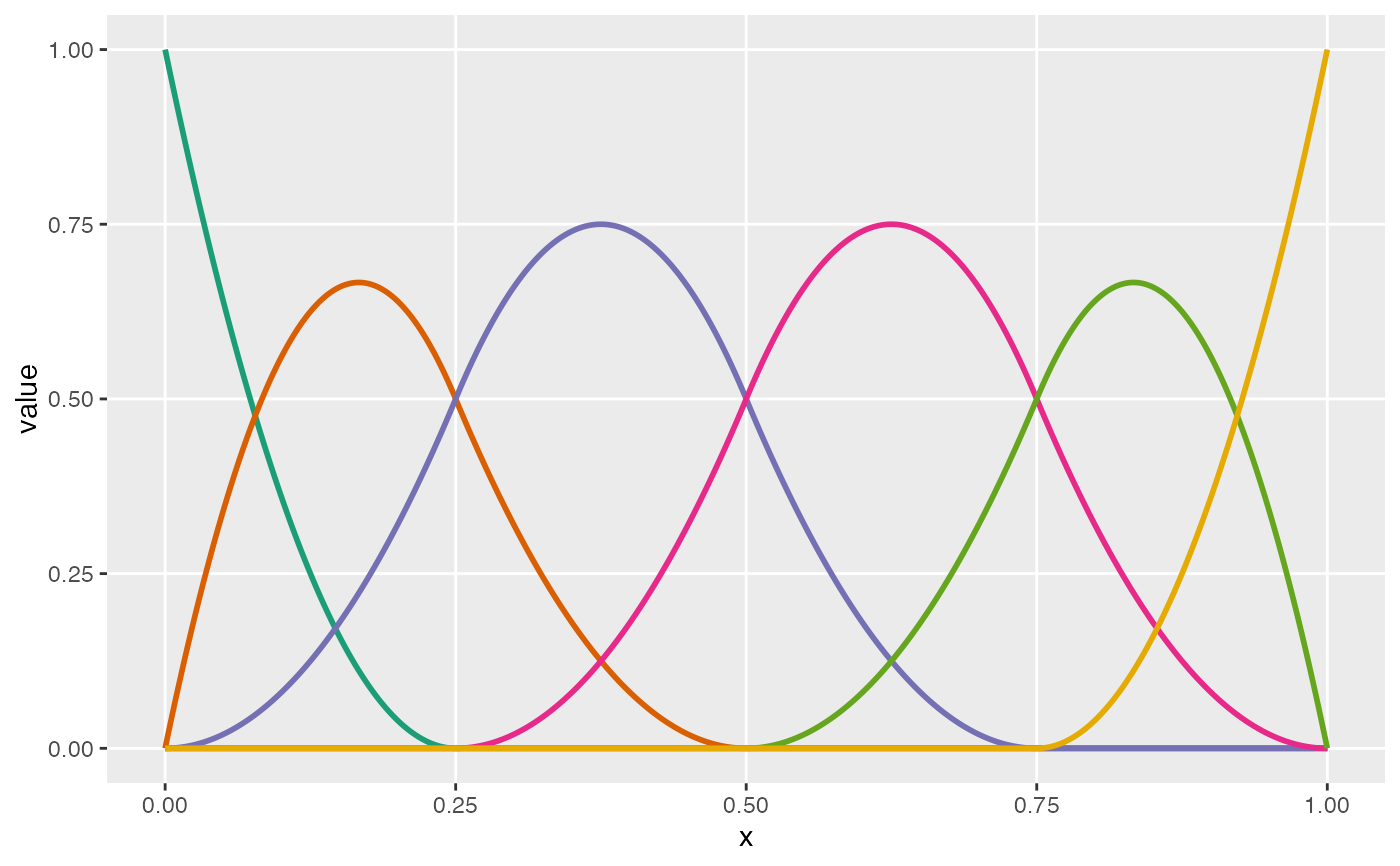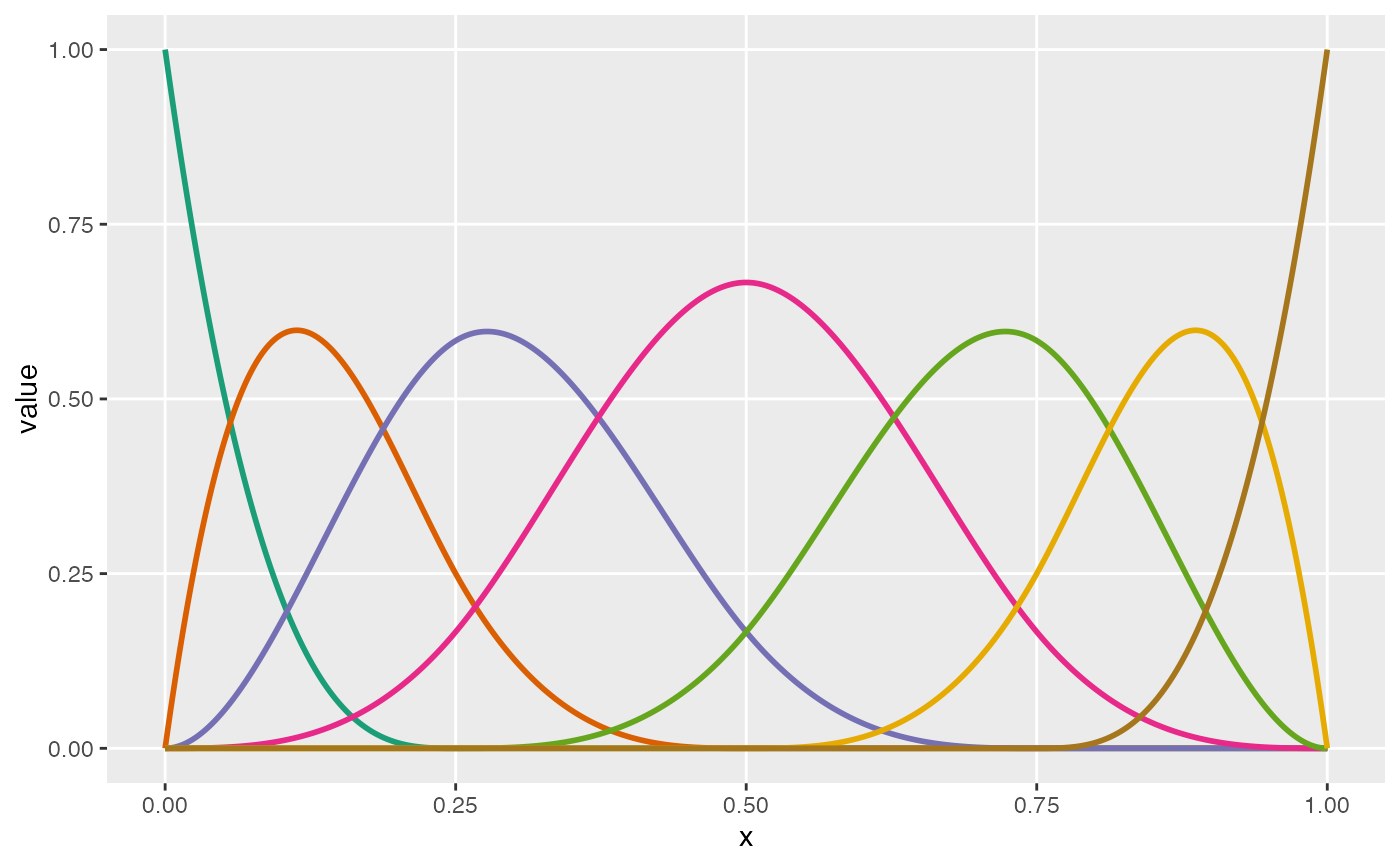Plot basis spline functions

viewBasis(knots, degree)

## Arguments

knots

A vector of values between 0 and 1, specifying cut-points for splines

degree

Integer specifying degree of curvature.

## Value

A ggplot object that contains a plot of the basis functions. In total, there will be length(knots) + degree + 1 functions plotted.

## Examples

knots <- c(0.25, 0.50, 0.75)
viewBasis(knots, degree = 1)knots <- c(0.25, 0.50, 0.75)
viewBasis(knots, degree = 2)knots <- c(0.25, 0.50, 0.75)
viewBasis(knots, degree = 3)